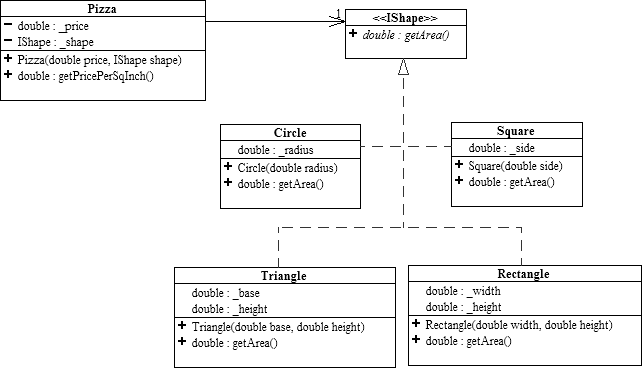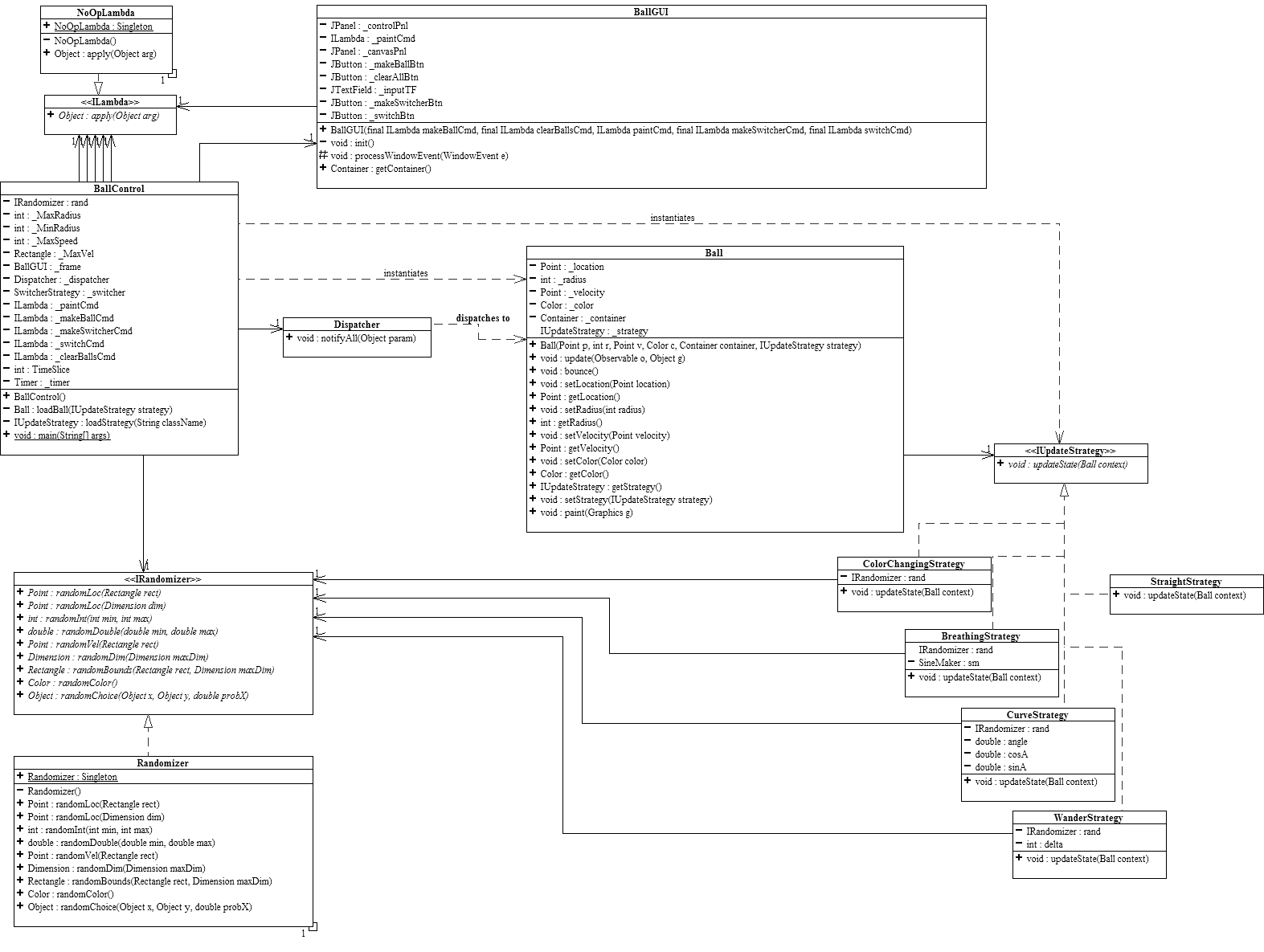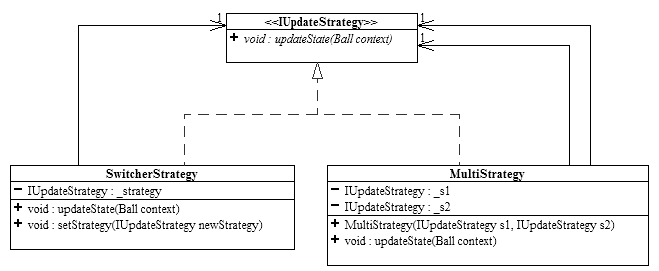# Ballworld: Composition-based

(Back to Connexions Modules Home)

WARNING: This Ballworld system described here uses an older style of the Model-View-Controller design pattern that the course no longer uses!

DO NOT ROTE COPY THE ARCHITECTURE DEPICTED HERE!! -- CONSULT YOUR CURRENT COURSE AS TO THE STYLE OF MVC ARCHITECTURE YOU ARE EXPECTED TO USE!

Specifically, following changes are made in later versions of Ballworld:

• `BallControl` is misleadingly named below, as it is actually the "model" portion of the MVC architecture and should be named accordingly.
• `ILambda` is replaced with more general, richer interfaces that are specifically designed to adapt the model to the view and vice versa.

Summary: The module uses the Ballworld program to demonstrate key ideas in abstract classes, polymorphism, inheritance and other coding techniques. In particular, this module will contrast a composition-based architecture with an inheritance-based architecture.

In this module we will explore what is gained by modifying the inheritance-based Ballworld system into a composition-based system.

In the inheritance-based Ballworld system, we were able to generate quite a bit of flexibility and extensibility. For instance, we could develop new kinds of balls and add them into the system without recompiling the rest of the code. This was accomplished by having the invariant ball creation and management code deal only with the abstract ball while the variant behaviors were encapsulated into the concrete subclasses.

## The Problem with Inheritance

Inheritance seems to work quite well, but suppose we want to do more than just put different kinds of balls on the screen? What if we wanted to be able to change how a ball behaves, after it has been created? What if we want create balls that do a multiple of different behaviors, such as change color and radius? While working solutions using an inheritance-based system do exist, they are cumbersome, inefficient and most importantly, inconsistent with any sort of clear abstract model of what balls should be like.

The problem lies in the very nature of inheritance. When we attempted to separate the variant from the invariant behaviors, we overlooked a crucial aspect of inheritance. In our model, the superclass represented the invariant behaviors of a ball while the subclasses represented the variant behaviors. The separation seemed clear enough in the UML diagram, except that when one has an actual object instanceboth the superclass and subclass behaviors are bound into a single entity. A ball object cannot change its variant updateState behavior because it is inextricably bound with to the invariant behaviors. A ball object cannot be composed of multiple updateState behaviors because that code cannot be isolated from the rest of the ball's code. If you want a curving behavior, you have to get it packaged in a whole ball object--you can't get just the behavior.

A clear sympton of this problem is the common code to call the superclass constructor found in all the subclasses' constructors. This tells us that the superclass is really right there in the subclass with everything else. The fact that the code is repeated from class to class says that it is invariant code in the middle of what we want to be variant code.

The inheritance-based model of Ballworld does not separate the variant and the invariant at the proper place. There is invariant code mixed together with the variant code.
That's why they can't be separated and the invariant behaviors are dragged along with the variant behaviors. This is what makes dynamically changing behaviors and multiply composed behaviors so difficult in this system.

## Pizzas and Shapes

To understand what we can do to remedy the problems with our inheritance-based model, let's digress for a bit and consider a simple model of pizzas. Here, we have a pizza which has a price and has a shape. A shape, be it a circle, square, rectangle of triangle, is capable of determining its own area. A pizza, when requested to calculate its price per square inch, simply takes its price and divides it by the area of its shape. To obtain that area, the Pizza delegates to the IShape, since it is the shape that knows how to calculate its area, not the pizza.

Figure 1: A pizza has-a shape, which is able to calculate its area.
Pizzas and ShapesDelegation is the handing of a calculation off to another object for it process. Here, the pizza is only interested in the result of the area calculation, not how it is performed.
To the pizza, the shape represents an abstract algorithm to calculate the area.
The Pizza and the IShape classes represent the invariant processes involved with calculating the price per square inch ration, while the concrete Circle, Square, Triangle and Rectangle classes represent the variant area calculations for different shapes. What wee see from this example is that
objects can be used to represent pure behavior, not just tangible entities.
Interfaces are particularly useful here as they are expressly designed to represent pure, abstract behavior.

## From Inheritance to Composition

Coming back to Ballworld, we see that the updateState method in ABall is an abstract algorithm to update the state of the ball. So, just as in the pizza example, we can represent this algorithm, and just this algorithm, as an object. We can say that a ball has an algorithm to update its state. Another wa of saying this is to say that the ball has a strategy to update its state. We can represent this by using composition. Instead of having an abstract method to update the state, we model a ball as having a reference to an IUpdateStrategy object. The code for update thus becomes

```  	public void update(Observable o, Object g)
{
_strategy.updateState(this);   // update this ball's state using the strategy
location.translate (velocity.x, velocity.y);  // move the ball
bounce();  // bounce the ball off the wall if necessary
paint((Graphics) g); // paint the ball onto the container
}```
The ball hands a reference to itself, this, to the strategy so that the strategy knows which ball to update. The variant updating behaviors are now represented by concrete implementations of the IUpdateStrategy interface.
Figure 2: Ball is now a concrete class and its subclasses have been eliminated in favor of being composed with an abstract strategy.
Composition-based Ballworld(Note that the Randomizer class has been redesigned to eliminate its static methods. One new method has been added as well.)

• The modified ABall class now contains 100% concrete code and thus should not be abstract anymore.
• ABall has been renamed to simply Ball.
• Accessor methods for the strategy (getStrategy and setStrategy) have been added.
• The Ball class is still 100% invariant code.
• The CurveBall, StraightBall, etc. subclasses are no longer needed as their variant behaviors have been moved to the IUpdateStrategy subclasses.
• Effectively what has happened is that the updateState method has been moved from the ABall subclasses and embodied into their own classes.
• The IUpdateStrategy subclasses do not inherit anything from Ball, hence they do not contain any invariant code.
• The strategies are thus 100% variant code.
• The reference to the ball during the updating process has gone from a peristent communication link (implicitly, this), to a transient communication link (host).
• This composition-based model divides the code exactly between the variant and invariant behaviors--this is the key to the power and flexibility it generates.

This new composition-based model of Ballworld is an example of the Strategy Design Pattern. The strategy design pattern allows us to isolate variant behaviors on a much finer level than simple inheritance models.

### Composing Behaviors

So far, all of our redesigning has resulted in a system that behaves exactly as it did when we started. But what one finds very often in developing systems is that in order to make two steps forward, one must first make one step backwards in order to fundmentally change the direction in which they are going. So, even though it looks like our system has not progressed because it still does exactly the same thing, we are indeed in a very different position, architecturally. By freeing the variant behaviors from the invariant ones, we have generated a tremendous amount of flexibility.

#### Balls that change their strategies

Let's consider a the notion of a ball that changes its behavior. Since we have modeled a ball as having a strategy, we can simply say that in some manner, it is the ball's strategy that changes. We could say that the ball changes its strategy, but since the ball doesn't know which strategy it has to begin with, it really doesn't know one strategy from another. One could argue that it therefore can't know when or if it should ever change its strategy. Therefore, the ball cannot be coded to change its own strategy! So, whose baliwick is the changing of the strategy?

Since the changing of a strategy is a strategy for updating the ball, it is the strategy that determines the change. The strategy changes the strategy! Let's consider the following strategy:

```	package ballworld;
import java.awt.*;

public class Change1Strategy implements IUpdateStrategy {

private int i = 500;  // initial value for i

if(i==0) context.setStrategy(new CurveStrategy());   // change strategy if i reaches zero
else i--;  // not yet zero, so decrement i
}
}```
This strategy acts just like a StraightStrategy for 500 updates and then it tells the ball (its context) to switch to a CurveStrategy. Once the CurveStrategy is installed, the ball becomes curving, without the need for any sort of conditionals to decide what it should do. The context ball fundamentally and permanently becomes curving.
##### EXERCISE 1

What would happen if you had two strategies like the one above, but instead of replacing themselves with CurveStrategy's , they instead instantiated each other?

###### SOLUTION

Try it!

A key notion above is that a strategy can contain another strategy. In the above example, the Change1Strategy could have easily pre-instantiated the CurveStrategy and saved it in a field for use when it was needed. But the does it matter exactly which concrete strategy is being used? If not, why not work at a higher abstraction level and let one strategy hold a reference to an abstract strategy? For instance, consider the following code:

```	package ballworld;
import java.awt.*;

public class SwitcherStrategy implements IUpdateStrategy {

private IUpdateStrategy _strategy = new StraightStrategy();

}

_strategy = newStrategy;
}
}```
This strategy doesn't look like it does much, but looks are deceiving. All the SwitcherStrategy does is to delegate the updateState method to the _strategy that it holds. This does not seem much in of itself, but consider the fact that the SwitcherStrategy also has a settor method for _strategy. This means that the strategy held can be changed at run time! More importantly, suppose a ball is instantiated with a SwitcherStrategy. The behavior of the ball would be that of whatever strategy is being held by the SwitcherStrategy since the switcher just delegates to the held strategy. If one were to have a reference to that SwitcherStrategy instance from somewhere else, one could then change the internal strategy. The ball is none the wiser because all it has is a reference to the SwitcherStrategy instance, which hasn't changed at all! However, since the held strategy is now different, the ball's behavior has completely changed! This is an example of the Decorator Design Pattern, where the SwitcherStrategy class is formally called the decorator and the held strategy is formally called the decoree. In theoretical terms, the decorator is what is known as an indirection layer, which is like a buffer between two enities that enables them to depend on each other but yet still be free to move and change with respect to each other. A very useful analogy for indirection layers is like the thin layer of oil that will enable two sheets of metal to slide easily past each other.

#### Balls with multiple, composite behaviors

Now that we can dynamically change a ball's behavior, let's tackle another problem:

##### EXERCISE 2

How can we have balls with multiple behaviors but yet not duplicate code for each one of those behaviors?

###### SOLUTION

Use the same techniques as before: strategies that hold strategies.

```	package ballworld;
import java.awt.*;

public class DoubleStrategy implements IUpdateStrategy {

private IUpdateStrategy _s1 = new CurveStrategy();
private IUpdateStrategy _s2 = new BreathingStrategy();

}
}```
Ta da! No problem. The DoubleStrategy simply holds two strategies and delegates to each of them in turn when asked to updateState. So why stop here?
```	package ballworld;
import java.awt.*;

public class TripleStrategy implements IUpdateStrategy {

private IUpdateStrategy _s1 = new CurveStrategy();
private IUpdateStrategy _s2 = new BreathingStrategy();
private IUpdateStrategy _s3 = new BreathingStrategy();

}
}```
We're on a roll now! We could go on and on, making as complex a strategy as we'd like, making a new class for each combination we want. But somewhere around the 439'th combination, we get mightly tired of writing classes. Isn't there an easier way?

Abstraction is the key here. We want to write code that represents that abstraction of multiple, composite strategies. Does what we were doing above depend on the particular concrete strategies that we were using? No? Then we should eliminate the concrete classes, raise the abstraction level and use the abstract superclass (interface) instead. For a combination of two behaviors, we end up with the following:

```	package ballworld;
import java.awt.*;

public class MultiStrategy implements IUpdateStrategy {

_s1 = s1;
_s2 = s2;
}

}
}```
Notice how we have added a constructor that enables us to initialize the two abstract strategy fields. All we have to do is to construct a MultiStrategy object with the two desired strategies, and we're good to go!

##### EXERCISE 3

So if we want three behaviors, all we have to do is to make the same sort of thing but with 3 abstract strategy fields, right?

###### SOLUTION

But isn't a MultiStrategy an IUpdateStrategy iteself? That is, since a MultiStrategy holds IUpdateStrategy's, couldn't a Multistrategy hold a Multistrategy , which is holding a Multistrategy (or two) which is hold a Multistrategy , which is holding.....?

Thus, with just a Multistrategy we are capable of composing arbitrarily complex behaviors!

#### Composite Patterns

So what have we wrought here? Let's take a look at the UML diagram of our to most abstract strategies.

Figure 3: Note that the subclasses hold references to their own superclasses!
SwitcherStrategy and MultiStrategyThe key to the power that lies in the SwitcherStrategy and the MultiStrategy lies in the fact that they hold references to their own superclass, IUpdateStrategy. This is what enables them to be create any behavior they want, including combinations of behaviors and dynamic modifications of behaviors. This self-referential class structure is known as the Composite Design Pattern (The Decorator pattern can be considered to be specialized form of the Composite pattern). The massive power, flexibility and extensiblility that this pattern generates warrants further, more formal study, which is where we're heading next. Stay tuned!

Originally published in Connexions (CNX): https://web.archive.org/web/20130326221559/http://cnx.org/content/m11816/latest/